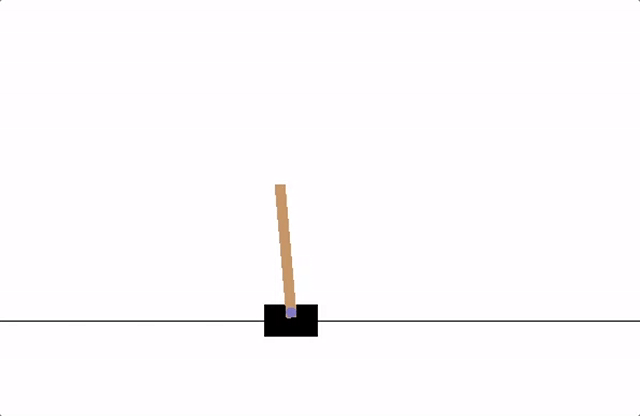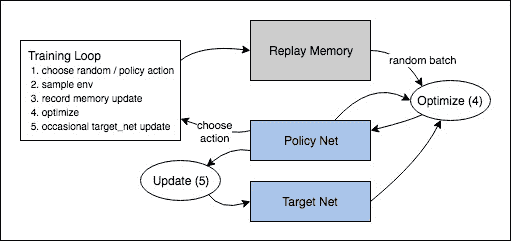# 强化学习CartPole任务的设计是，代理人的输入是4个表示环境状态的实数（位置、速度等）。我们不进行任何缩放地获取这4个输入，并将它们通过一个具有2个输出的小型全连接网络，每个动作一个输出。该网络经过训练以预测给定输入状态下每个动作的期望值。然后选择期望值最高的动作。

## 库

%%bash
pip3 install gymnasium[classic_control]


• 神经网络 (torch.nn)

• 优化 (torch.optim)

• 自动微分 (torch.autograd)

import gymnasium as gym
import math
import random
import matplotlib
import matplotlib.pyplot as plt
from collections import namedtuple, deque
from itertools import count

import torch
import torch.nn as nn
import torch.optim as optim
import torch.nn.functional as F

env = gym.make("CartPole-v1")

#建立matplotlib
is_ipython = 'inline' in matplotlib.get_backend()
if is_ipython:
from IPython import display

plt.ion()

# 如果用GPU的话
device = torch.device("cuda" if torch.cuda.is_available() else "cpu")


## 回放内存

• Transition - 一个命名的元组，表示我们环境中的单个转换。它基本上将(状态、动作）对映射到它们的(下一个状态、奖励）结果，状态是屏幕差分图像，如后面所述。

• ReplayMemory -一个有界大小的循环缓冲区，用于保存最近观察到的转换。它还实现了一个.sample(）方法，用于选择一批随机转换进行训练。

Transition = namedtuple('Transition',
('state', 'action', 'next_state', 'reward'))

class ReplayMemory(object):

def __init__(self, capacity):
self.memory = deque([], maxlen=capacity)

def push(self, *args):
"""保存转换"""
self.memory.append(Transition(*args))

def sample(self, batch_size):
return random.sample(self.memory, batch_size)

def __len__(self):
return len(self.memory)


## DQN 算法

Q-Learning背后的主要思想是，如果我们有一个函数 $$Q^*: State \times Action \rightarrow \mathbb{R}$$, 则如果我们在特定的状态下采取行动，那么我们可以很容易地构建一个最大化回报的策略：

$$\pi^*(s) = \arg\!\max_a \ Q^*(s, a)$$

$$\delta = Q(s, a) - (r + \gamma \max_a Q(s', a))$$

$$\mathcal{L} = \frac{1}{|B|}\sum_{(s, a, s', r) \ \in \ B} \mathcal{L}(\delta)$$

$$\begin{split} \text{where} \quad \mathcal{L}(\delta) = \begin{cases} \frac{1}{2}{\delta^2} & \text{for } |\delta| \le 1, \ |\delta| - \frac{1}{2} & \text{otherwise.} \end{cases} \end{split}$$

## Q-网络

class DQN(nn.Module):

def __init__(self, n_observations, n_actions):
super(DQN, self).__init__()
self.layer1 = nn.Linear(n_observations, 128)
self.layer2 = nn.Linear(128, 128)
self.layer3 = nn.Linear(128, n_actions)

# 使用一个元素调用以确定下一个动作，或者在优化期间使用一批
# 返回 tensor([[left0exp,right0exp]...])。
def forward(self, x):
x = F.relu(self.layer1(x))
x = F.relu(self.layer2(x))
return self.layer3(x)


## 训练

### 超参数和配置

• select_action - 将根据迭代次数贪婪策略选择一个行动。简单地说，我们有时会使用我们的模型来选择动作，有时我们只会对其中一个进行统一的采样。选择随机动作的概率将从 EPS_START 开始并以指数形式向 EPS_END衰减。 EPS_DECAY 控制衰减速率。
• plot_durations - 一个帮助绘制迭代次数持续时间，以及过去100迭代次数的平均值(官方评估中使用的度量）。迭代次数将在包含主训练循环的单元下方，并在每迭代之后更新。
# BATCH_SIZE 是从重播缓冲区采样的转换数量
# GAMMA 是前一节中提到的折扣因子
# EPS_START 是 epsilon 的起始值
# EPS_END 是 epsilon 的最终值
# EPS_DECAY 控制 epsilon 指数衰减的速率，数值越高意味着衰减越慢
# TAU 是目标网络的更新速率
# LR 是 AdamW 优化器的学习率
BATCH_SIZE = 128
GAMMA = 0.99
EPS_START = 0.9
EPS_END = 0.05
EPS_DECAY = 1000
TAU = 0.005
LR = 1e-4

# 从 gym 动作空间获取动作数量
n_actions = env.action_space.n
# 获取状态观察的数量
state, info = env.reset()
n_observations = len(state)

policy_net = DQN(n_observations, n_actions).to(device)
target_net = DQN(n_observations, n_actions).to(device)

memory = ReplayMemory(10000)

steps_done = 0

def select_action(state):
global steps_done
sample = random.random()
eps_threshold = EPS_END + (EPS_START - EPS_END) * \
math.exp(-1. * steps_done / EPS_DECAY)
steps_done += 1
if sample > eps_threshold:
# t.max(1）将为每行的列返回最大值。max result的第二列是找到max元素的索引，因此我们选择预期回报较大的操作。
return policy_net(state).max(1).view(1, 1)
else:

episode_durations = []

def plot_durations(show_result=False):
plt.figure(1)
durations_t = torch.tensor(episode_durations, dtype=torch.float)
if show_result:
plt.title('Result')
else:
plt.clf()
plt.title('Training...')
plt.xlabel('Episode')
plt.ylabel('Duration')
plt.plot(durations_t.numpy())
# 平均 100 次迭代画一次
if len(durations_t) >= 100:
means = durations_t.unfold(0, 100, 1).mean(1).view(-1)
means = torch.cat((torch.zeros(99), means))
plt.plot(means.numpy())

plt.pause(0.001)  # 暂定一会等待屏幕更新
if is_ipython:
if not show_result:
display.display(plt.gcf())
display.clear_output(wait=True)
else:
display.display(plt.gcf())


### 训练循环

def optimize_model():
if len(memory) < BATCH_SIZE:
return
transitions = memory.sample(BATCH_SIZE)
# 转置批样本(有关详细说明，请参阅https://stackoverflow.com/a/19343/3343043）。这会将转换的批处理数组转换为批处理数组的转换。
batch = Transition(*zip(*transitions))

# 计算非最终状态的掩码并连接批处理元素(最终状态将是模拟结束后的状态）
non_final_mask = torch.tensor(tuple(map(lambda s: s is not None,
batch.next_state)), device=device, dtype=torch.bool)
non_final_next_states = torch.cat([s for s in batch.next_state
if s is not None])
state_batch = torch.cat(batch.state)
action_batch = torch.cat(batch.action)
reward_batch = torch.cat(batch.reward)

# 计算Q(s_t, a)-模型计算 Q(s_t)，然后选择所采取行动的列。这些是根据策略网络对每个批处理状态所采取的操作。
state_action_values = policy_net(state_batch).gather(1, action_batch)

# 计算下一个状态的V(s_{t+1})。非最终状态下一个状态的预期操作值是基于“旧”目标网络计算的；选择max(1)的最佳奖励。这是基于掩码合并的，这样当状态为最终状态时，我们将获得预期状态值或0。
next_state_values = torch.zeros(BATCH_SIZE, device=device)
# 计算期望 Q 值
expected_state_action_values = (next_state_values * GAMMA) + reward_batch

# 计算 Huber 损失
criterion = nn.SmoothL1Loss()
loss = criterion(state_action_values, expected_state_action_values.unsqueeze(1))

# 优化模型
loss.backward()
# 原地梯度裁剪
optimizer.step()


if torch.cuda.is_available():
num_episodes = 600
else:
num_episodes = 50

for i_episode in range(num_episodes):
# 初始化环境和状态
state, info = env.reset()
state = torch.tensor(state, dtype=torch.float32, device=device).unsqueeze(0)
for t in count():
action = select_action(state)
observation, reward, terminated, truncated, _ = env.step(action.item())
reward = torch.tensor([reward], device=device)
done = terminated or truncated

if terminated:
next_state = None
else:
next_state = torch.tensor(observation, dtype=torch.float32, device=device).unsqueeze(0)

# 在内存中储存当前参数
memory.push(state, action, next_state, reward)

# 进入下一状态
state = next_state

# 执行优化的一步（在策略网络上）
optimize_model()

# 目标网络权重的软更新
# θ′ ← τ θ + (1 −τ )θ′
target_net_state_dict = target_net.state_dict()
policy_net_state_dict = policy_net.state_dict()
for key in policy_net_state_dict:
target_net_state_dict[key] = policy_net_state_dict[key]*TAU + target_net_state_dict[key]*(1-TAU)

if done:
episode_durations.append(t + 1)
plot_durations()
break

print('Complete')
plot_durations(show_result=True)
plt.ioff()
plt.show()